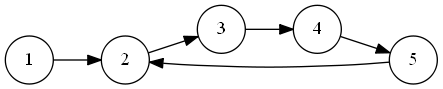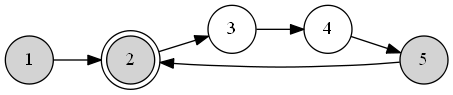# Cycle Detection With Floyd Tortoise And HareMar 6th, 2019 - written by Kimserey with .

Floyd’s Tortoise and Hare is a cycle detection algorithm operating on a linked list. The algorithm is based on two pointers, the tortoise and the hare, moving on the linked list at a different speed. The algorithm can be used to find cycle existence, deduces the beginning of the cycle, and the length of a cycle. Today we will explore the mathematical proof behind the algorithm and we will implement it in Racket.

## Floyd’s Tortoise and Hare

A linked list can be represented as a directed graph of nodes. Consider the following graph, with nodes going from one to five, where five is linking back to two creating a cycle.In this particular example, Floyd’s Tortoise and Hare can identity:

1. the existence of the cycle,
2. the starting index of the cycle,
3. the length of a cycle.

Consider $\mu$, the beginning of the cycle, $\lambda$ the length of a cycle, and $k$ a constant, for any $i>=\mu$ and $k>=0$, we have:

$(1)\quad x_{i} = x_{i + k\lambda}$

Since $\mu$ is the beginning of the cycle, the constraint $i>=\mu$ indicates that we are choosing indexes which are within the cycle. And from within the cycle, each index value is equal to the value at the $index + full\,iteration\,of\,the\,cycle$.

Following $(1)$, we will have $i=k\lambda$ if and only if $x_{i} = x_{2i}$. As in this case we have:

$\begin{split} (2)\quad 2i &= i + k\lambda \\ i &= k\lambda \end{split}$

Therefore if we find a point where $x_{i} = x_{2i}$ then $i$ will be a multiple of $\lambda$.

Finding this point can be implemented by two pointers, a $slow$ pointer and a $fast$ pointer, moving on the list by one element for $slow$ and two elements for $fast$ which, if a cycle exists, eventually meet at $x_{i} = x_{2i}$. We can be certain that $slow$ and $fast$ will meet as from the point of view of $slow$, $fast$ is moving one step at a time, therefore for each step $slow$ is taking, $fast$ get closer by one step.

We demonstrated that if $slow$ move one step at a time, and $fast$ move two steps at a time, if a cycle exists, they will eventually meet.

Here is the meeting point of our list:If we call $v$ the distance travelled by the $slow$ pointer till the meeting point, from $(2)$, we have $v=k\lambda$, and $v$ is a multiple of the length of the cycle $\lambda$.

Being a multiple of $\lambda$ indicates that the distance $v$ can be expressed as a multiple of the cycle.

$Fast$ travelling twice as fast as $slow$, $fast$ reaches $v$ first, and when $fast$ reaches $v$, $slow$ is at $\frac{v}{2}$. From here, $fast$ is already within the cycle, by the time $slow$ travels $\frac{v}{2}$ remaining, $fast$ will have done an iteration of the cycle of lentgh $\lambda$ and fall on the same point therefore we can conclude that $v=k\lambda$. In other words, the distance from the beginning of the list to the meeting point is divisible by the cycle length.

We demonstrated that when $slow$ and $fast$ meet, the distance $v$ is a multiple of the cycle $\lambda$.

From $(1)$ and $(2)$, we can deduce that for $i>=\mu$, we have:

$\begin{split} x_{i}&=x_{i+k\lambda}\\ x_{i}&=x_{k\lambda+i}\\ x_{i}&=x_{v+i}\\ \end{split}$

And therefore if we take $i=\mu$, we will get:

$x_{\mu}=x_{v+\mu}$

The length from the beginning of the list till the root of the cycle $\mu$ is equal to the length from the meeting point $v$ to the root of the cycle $v+\mu$.

Therefore, continuing with $slow$ and $fast$ previously at $v$, we place back $fast$ to the beginning of the list, and advance both of them at same speed, at $\mu$, we will have $slow=x_{\mu}$ and $fast=x_{v+\mu}$.

We demonstrated that by placing $fast$ to the beginning, and keeping $slow$ at the meeting point, and moving $slow$ and $fast$ at the same speed, they will meet again at the beginning of the cycle.Lastly from $\mu$, we can move $slow$ to complete a full cycle and count the number of steps to know the length of a cycle.Let’s see now how can the algorithm be implemented in Racket.

## Implementation

For this algorithm, we know that we will need the following functionalities:

1. a next function, to find the next node in the linked list,
2. a meet function, to traverse the list for the tortoise and hare, and find the meeting point,
3. a way to count the iterations to deduce the cycle length.

We start first by defining a list of nodes.

1
2
3
4
5
6
7
(define nodes
(list
(cons 1 2)
(cons 2 3)
(cons 3 4)
(cons 4 5)
(cons 5 2)))


Then using the nodes, we create a function next which finds the child by recursively looking through the nodes.

1
2
3
4
5
6
(define (next parent)
(define (find nodes parent)
(match nodes
[(list (cons p c) rest ...) #:when (= parent p) c]
[_ (find (cdr nodes) parent)]))
(find nodes parent))


We create next-next, which allows us to move twice on the list.

1
(define (next-next x) (next (next x)))


Then we create meet, a function traversing the list with two pointers, and returning the point where the pointers meet together with the number of iterations.

1
2
3
4
5
6
7
(define (meet slow fast p1 p2 steps)
(let ([p1 (slow p1)]
[p2 (fast p2)]
[steps (+ 1 steps)])
(cond
[(= p1 p2) (cons p1 steps)]
[else (meet slow fast p1 p2 steps)])))


We then create utils to return the result, or the count.

1
2
3
4
5
(define (meet/result slow fast p1 p2)
(car (meet slow fast p1 p2 0)))

(define (meet/count slow fast p1 p2)
(cdr (meet slow fast p1 p2 0)))


Lastly we create a find-cycle, a function which as describes by the steps in the first section starts by finding the meeting point. Then from the meeting point finds the root. Then from the root finds the lenght of the cycle.

1
2
3
4
5
6
7
8
9
10
11
12
13
14
15
16
17
18
19
(define (find-cycle init)

; Step 1: Traverse the list with tortoise 1x and hare 2x
; to find meeting point.
(define cycle-meet
(list-traverse/result next next-next init init))

; Step 2: Move hare back to beginning and traverse at
; same speed 1x to find cycle root.
(define cycle-root
(list-traverse/result next next cycle-meet init))

; Step 3: Keep tortoise in place and move hare
; on the cycle at 1x to execute one full circle and find
; the length.
(define cycle-length
(list-traverse/count identity next cycle-root cycle-root))

(list cycle-meet cycle-root cycle-length))


We can then run find-cycle providing an initial point:

1
2
> (find-cycle 1)
'(5 2 4)


Starting from one, the meeting point of our linked list is five, the root is two and the cycle length is four.

## Python Implementation

The same implementation can be achieved in python where we define:

1. next to get the next link,
2. next_next to get the next next link,
3. meet to calculate the meeting point,
4. meet_result and meet_count to extract the meeting point and the count of steps to reach the meeting point,
5. find_cycle, the main function finding the cycle.
1
2
3
4
5
6
7
8
9
10
11
12
13
14
15
16
17
18
19
20
21
22
23
24
25
26
27
28
29
30
31
32
33
34
35
36
37
38
39
nodes = [(1, 2), (2, 3), (3, 4), (4, 5), (5, 2)]

def next(parent):
def find(nodes, parent):
current = nodes
rest = nodes[1:]

if current and current == parent:
return current
else:
return find(rest, parent)
return find(nodes, parent)

def next_next(x):
return next(next(x))

def meet(slow, fast, p1, p2, steps):
p1 = slow(p1)
p2 = fast(p2)
steps = steps + 1

if p1 == p2:
return (p1, steps)
else:
return meet(slow, fast, p1, p2, steps)

def meet_result(slow, fast, p1, p2):
result = meet(slow, fast, p1, p2, 0)
return result

def meet_count(slow, fast, p1, p2):
result = meet(slow, fast, p1, p2, 0)
return result

def find_cycle(init):
cycle_meet = meet_result(next, next_next, init, init)
cycle_root = meet_result(next, next, cycle_meet, init)
cycle_length = meet_count(lambda x: x, next, cycle_root, cycle_root)
return (cycle_meet, cycle_root, cycle_length)


And it can be used by calling the following:

1
2
print(find_cycle(1))
> (5, 2, 4)


And that concludes today’s post!

## Conclusion

When I first encountered the Floyd’s Tortoise and Hare I was totally puzzled by its simplicity, yet it never failed to work, for any sort of linked-list I could come up with. I couldn’t understand why the tortoise and hare would always meet a second time at the root of the cycle. This was truly amazing to me, and is the reason why I wanted to write a deeper explanation, mixed with my thoughts, in this blog post.

Today we saw how Floyd’s Tortoise and Hare algorithm works. We started by verifying the mathematical proof demonstrating the validity of the algorithm. It is truly amazing how without Then we moved to implement it with Racket. I hope you liked this post, and I see you on the next one!

## External Sources

Designed, built and maintained by Kimserey Lam.